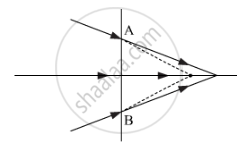Share

# The Line Ab in the Ray Diagram Represents a Lens State Whether the Lens is Convex Or Concave. - CBSE (Science) Class 12 - Physics

#### Question

The line AB in the ray diagram represents a lens State whether the lens is convex or concave.#### Solution

The lens is concave in nature as it slightly diverges the path of the refracted light.

Is there an error in this question or solution?

#### Video TutorialsVIEW ALL 

Solution The Line Ab in the Ray Diagram Represents a Lens State Whether the Lens is Convex Or Concave. Concept: Lenses.
S